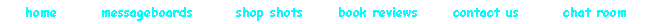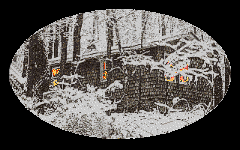Compound Miter Formula
by David Wright

 Miter angle tables are handy for many common situations, but they don't help much when your designs call for unusual angles.  Trial and error fitting approaches can drive you crazy.  Now is the right time to get a cup of coffee and pull out a calculator with trig functions.  Here are the formulas that were used to make up those handy tables.  Refer to the sample diagram as you read the variable descriptions, formulas, and the sample diagram solution.Variable Descriptions A = Angular bend that the corner makes relative to a straight line.  Not that it matters, but A = 0 for a straight line.  As for useful angles, A = 90 for a square corner, A = 60 for a hexagon, and A = 45 for an octagon. B = tilt angle of each workpiece away from the base plane.  B = 45 degrees for the sample diagram. X = crosscut angle for each piece; this is the setting for your table saw miter gauge or SCMS turntable.  X = 0 for a square cut.  You will have to use the complement of X if your saw labels a square cut as 90 degrees. Y = blade tilt for each piece.  Y = 0 for a square cut.  You will have to use the complement of Y if your saw labels a square cut as 90 degrees. Formulas X = arctan(cos(B) * tan(A/2)) Y = arcsin(sin(B) * sin(A/2)) Sample Diagram Solution ```* X = arctan(cos(B) * tan(A/2)) = arctan(cos(45) * tan(90/2)) = arctan(cos(45) * tan(45)) = arctan(0.7071 * 1) = arctan(0.7071) = 35.26 degrees * Y = arcsin(sin(B) * sin(A/2)) = arcsin(sin(45) * sin(90/2)) = arcsin(sin(45) * sin(45)) = arcsin(0.7071 * 0.7071) = arcsin(0.5000) = 30.00 degrees * To make this cut, rotate your SCMS turntable to 35.26 degrees away from a square cut, and tilt the blade over 30 degrees from a square cut.``` These formulas will tell you how far to go, but you still have to ponder left, right, upside down, and right side up.  Plenty of firewood opportunities even with the correct angles in hand.  I'm not a math whiz, so let me know if you have problems.  I copied them from my father (who is a math whiz); he made a polite comment about my having learned this in high school... Happy cutting, Dave CLICK HERE to download an automatic calculator spreadsheet, created by Joe Allred.Top